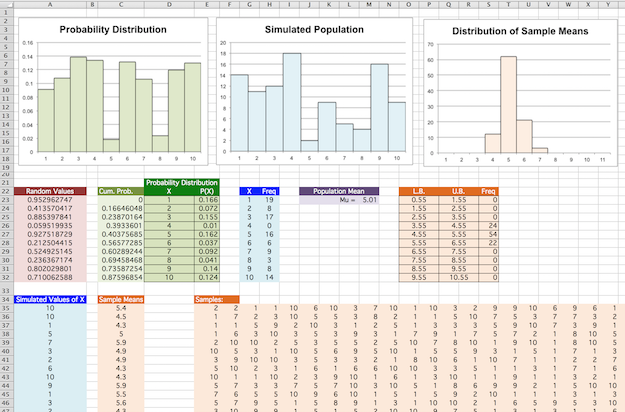## Excel Project: The Distribution of Sample Means

You are interested in the nature of the distribution of sample means when the samples in question come from a population that is decidedly non-normal in its distribution, and decide to model this all in Excel.

In an attempt to generate a very non-normal population, you decide to first produce a very non-normal probability distribution for a random variable X with possible integer outcomes from 1 to 10, inclusive. Then, you can use this probability distribution to generate a population of whatever size you wish.

1. To this end, on a blank worksheet, create a range of 10 random values from 0 to 1. For easy identification of these values, make the background fill color for this range light red (as shown in the image at the bottom of this page).

2. Of course, these values can't be used as probabilities for our outcomes 1 through 10, as they don't sum to 1. However, the fractions they represent of the value to which they do sum can be used as such probabilities. Use this fact to create a table to describe the related probability distribution, and make the background fill color for this table a medium green color (again, as shown in the image at the bottom of this page).

3. Create a probability histogram for this distribution, entitled "Probability Distribution", coloring the bars of the histogram light green (as shown).

4. It will be useful, momentarily, to have the cumulative probabilities up to each outcome $x$, (i.e., the sum $P(0) + P(1) + P(2) + \cdots + P(x-1)$ for any outcome $x$). With this in mind, add a column TO THE LEFT of the green range that defines your probability distribution that gives these cumulative probabilities. Make the background fill color for this column pale green (as shown).

5. Now, use these cumulative probabilities and the VLOOKUP() function to generate (in a single column) 100 simulated outcomes for $X$ tied to this probability distribution. Hint: pay particular attention to the 4th optional argument to the VLOOKUP() function, as you do this. These values will constitute the population from which you are drawing samples. Make the background fill color for this column pale blue (as shown, with label "Simulated Values of X").

6. Create a frequency table describing how often each $x$ value appears in your simulated population, also colored light blue (as shown, with labels "X" and "Freq")

7. Use this frequency table to make a histogram for the entire simulated population, with bars colored light blue, and entitled "Simulated Population".

8. Now create 100 samples, one per row, of 30 randomly selected members from your population, with means rounded to one decimal place for each in a separate column entitled "Sample Means". Both the samples and the sample means column should be colored light orange (as shown). You may find the INDEX() and ROUND() functions useful to this end.

9. Then create a frequency table for how often these means land in the classes $0.55$ to $1.55$, $1.55$ to $2.55$, $2.55$ to $3.55$, etc. (also colored orange, with column headers "L.B.", "U.B.", and "Freq"), and use it to create a histogram of the sample means you found.

10. Finally, calculate the population mean for the population generated, coloring it lilac.

11. Generate new populations, samples, and pictures by repeatedly pressing F9. What do you see? Which of the distributions look similar to one another? Do the distributions of sample means that result have a common shape? Where are the centers of the distributions of sample means located? How does the spread of the distributions of sample means compare to the spread of the population?